# How to reset index after Groupby pandas?

• Last Updated : 11 Dec, 2020

Python’s groupby() function is versatile.  It is used to split the data into groups based on some criteria like mean, median, value_counts, etc. In order to reset the index after groupby() we will use the reset_index() function.

Below are various examples which depict how to reset index after groupby() in pandas:

Attention geek! Strengthen your foundations with the Python Programming Foundation Course and learn the basics.

To begin with, your interview preparations Enhance your Data Structures concepts with the Python DS Course. And to begin with your Machine Learning Journey, join the Machine Learning - Basic Level Course

Example 1

## Python3

 `# import required modules``import` `numpy as np``import` `pandas as pd`` ` `# creating dataframe``df ``=` `pd.DataFrame({``'Subject'``: [``'Physics'``, ``                               ``'Chemistry'``, ``                               ``'Maths'``], ``                   ``'Marks'``: [``4``, ``8``, ``5``]})`` ` `# grouping the data on the basis of ``# subject and mean of marks.``df_grouped ``=` `df.groupby([``'Subject'``]).mean()`` ` `# display dataset``df_grouped`

Output: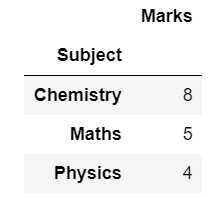Resetting the index after grouping data, using reset_index(), it is a function provided by python to add indexes to the data.

## Python3

 `# reset index``df_grouped.reset_index()`

Output: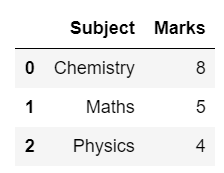Example 2:

Creating Dataframe.

## Python3

 `# import required modules``import` `pandas as pd``import` `numpy as np`` ` `# creating dataframe``df2 ``=` `pd.DataFrame({``'Student'``: [``1``, ``2``, ``3``, ``4``, ``1``, ``3``, ``2``, ``4``, ``1``, ``2``, ``4``, ``3``],``                    ``'Amount'``: [``                   ``10``, ``20``, ``30``, ``40``, ``20``, ``60``, ``40``, ``80``, ``30``, ``60``, ``120``, ``90``]})`` ` `# grouping the data``df2_group ``=` `df2.groupby([``'Student'``])`` ` `# grouped on the basis of students and``# with the value of count of amount``df2_grouped ``=` `df2_group[``'Amount'``].value_counts()`` ` `# display dataset``print``(df2_grouped)`

Output: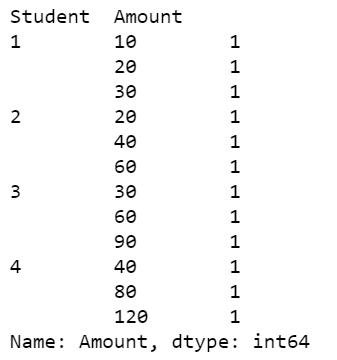Resetting the index. This will give you an error.

## Python3

 `# this will generate an error.``df2_grouped.reset_index()`

Output: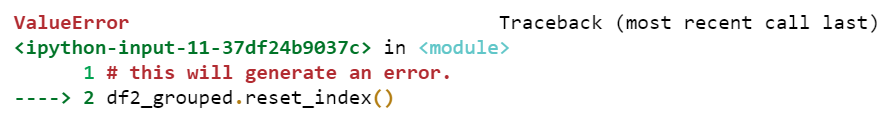Naming the reset_index() will group and reset the index.

## Python3

 `# resetting index on the basis of count``df2_grouped.reset_index(name ``=` `'count'``)`

Output: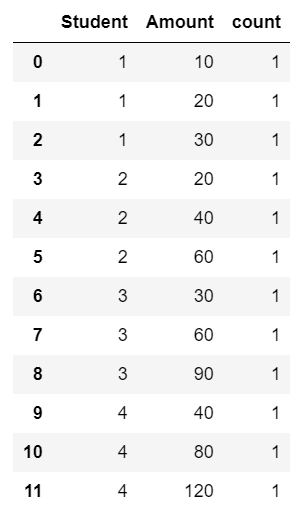Example 3

Here, is another example to depict how to reset dataframe after using groupby().

## Python3

 `# import required modules``import` `numpy as np``import` `pandas as pd`` ` `# creating dataframe``df ``=` `pd.DataFrame({``'Subject'``: [``'B'``, ``                               ``'C'``, ``                               ``'A'``,``'D'``,``'C'``,``'B'``,``'A'``], ``                   ``'Marks'``: [``4``, ``8``, ``5``,``9``,``8``,``1``,``0``]})`` ` `# grouping the data on the basis of ``# subject and mean of marks.``df_grouped ``=` `df.groupby([``'Subject'``]).mean()`` ` `# display dataset``df_grouped`` ` `# reset index``df_grouped.reset_index()`

Output: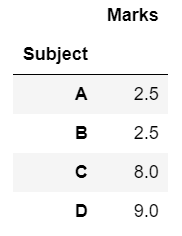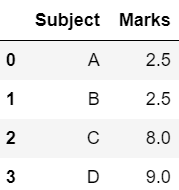My Personal Notes arrow_drop_up